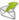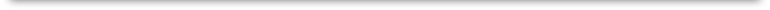Excel Math Blog
AnsMar Publishers, Inc.
12150 Tech Center Drive
Poway, CA 92064-7102
Toll Free: 866-866-7026
San Diego: 858-513-7900
Fax: 858-513-2764

info@excelmath.comTuesday, June 3, 2014

5 Steps to Solving Word ProblemsSome students struggle with solving word problems, not sure where to begin with finding the correct operation to use. Here are a few Excel Math tips to get your students started down the road to success: 1. Read the problem. It may help to read the problem aloud, just to make sure the students are reading it correctly. 2. Underline the important information. Use a red or blue pen to underline the information (or highlight it) so it doesn't get lost on the page: Jon picked one apple. Danny picked 5 apples. Together they picked how many apples?

3. What process will you use to get the answer:

Will you use addition, subtraction, multiplication, division, or a combination of all four?

Our apple example will use addition.

The key word here is together they picked how many?

4. Write and solve a number sentence. Then label the answer.

It may also help to draw a picture or use counters to represent the problem.

Here's a pictorial example of the apple word problem:The apple addition picture on the left gives us a good visual of the number sentence: 1 + 5 = 6 Your students could also use counters to the represent this equation. 5. Check to see if the answer makes sense. In this case, we know the answer should be more than 5, since Danny picked 5 apples. It may also help to do the reverse operation to check the answer. Since this word problem used addition, we subtract to check the answer: 6 - 1 = 5
 Here's a word problem to try out, using the steps shown above in red. 1. In this word problem, we start by reading the problem shown below. 2. The underlined information would be \$6.20, 3 quarters, 6 dimes and 9 nickels. 3. The process would include adding the change (after multiplying the amount of each coin) and then subtracting it from \$6.20:  3 x 25¢ = 75¢  6 x 10¢ = 60¢  9 x 5¢ = 45¢ The addition number sentence would be: 75¢ + 60¢ + 45¢ = 180 =  \$1.80 And the subtraction number sentence would be: \$6.20 - \$1.80 = \$4.40Excel Math word problem solution 4. So the answer to the word problem would be \$4.40. That means Luke has \$4.40 left over. 5. We can see at a glance that our answer is less than what Luke started with, so it is a reasonable answer. Finally, check the subtraction answer with addition (the reverse operation):  \$ 4.40 +  1.80   \$6.20 What are some additional tips you give your students to help them solve word problems? Leave a comment below.View Sample Lessons New to Excel Math? Visit our walk through tour to learn more and take a look at sample lessons: www.excelmath.com/tour/tour01.html Excel Math includes weekly and quarterly assessments along with a proven spiraling process and spaced repetition of concepts. With Excel Math, students build confidence in math and discover they can succeed. Walk through an Excel Math lesson here: http://excelmath.com/tour/tour03.html
 You may also enjoy these previous articles: May Day Teacher Appreciation Excel Math: TEKS Aligned and STAAR ReadyPowered byEMF Online HTML Form